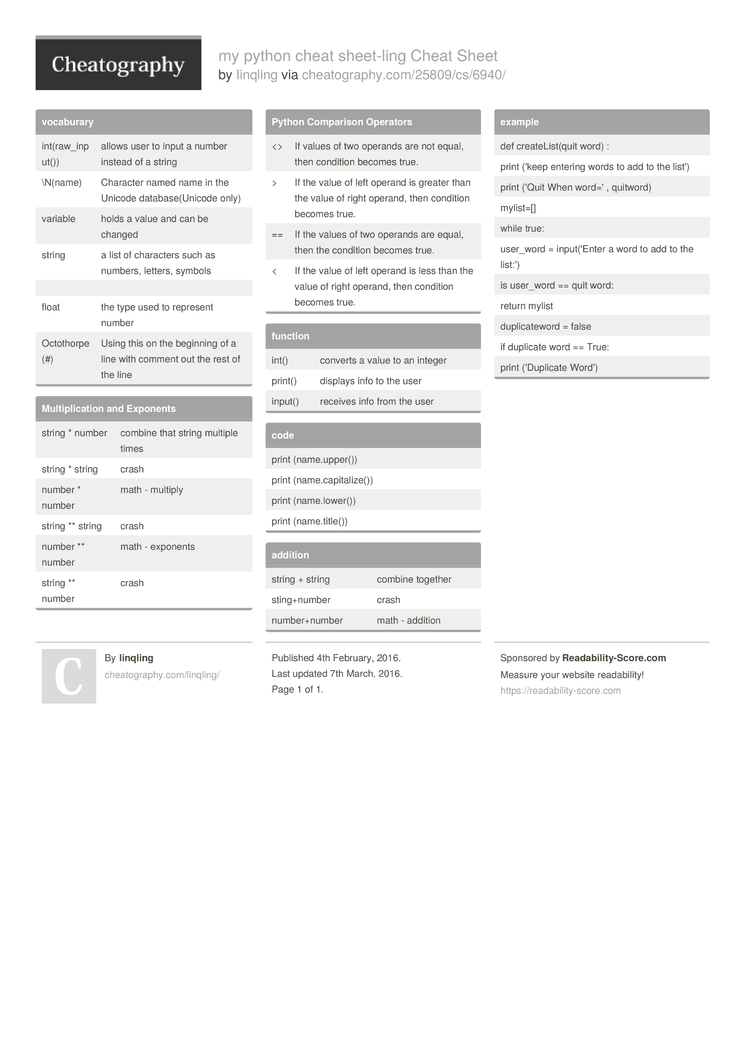Cheatography

# my python cheat sheet-ling Cheat Sheet by linqling

### vocaburary

 int(ra­w_i­nput()) allows user to input a number instead of a string \N(name) Character named name in the Unicode databa­se(­Unicode only) variable holds a value and can be changed string a list of characters such as numbers, letters, symbols float the type used to represent number Octothorpe (#) Using this on the beginning of a line with comment out the rest of the line

### Multip­­li­c­ation and Exponents

 string * number combine that string multiple times string * string crash number * number math - multiply string ** string crash number ** number math - exponents string ** number crash

### Python Comparison Operators

 <> If values of two operands are not equal, then condition becomes true. > If the value of left operand is greater than the value of right operand, then condition becomes true. == If the values of two operands are equal, then the condition becomes true. < If the value of left operand is less than the value of right operand, then condition becomes true.

### function

 int() converts a value to an integer print() displays info to the user input() receives info from the user

### code

 print (name.u­pp­er()) print (name.c­ap­ita­lize()) print (name.l­ow­er()) print (name.t­it­le())

 string + string combine together sting+­number crash number­+number math - addition

### example

 def create­Lis­t(quit word) : print ('keep entering words to add to the list') print ('Quit When word=' , quitword) mylist=[] while true: user_word = input(­'Enter a word to add to the list:') is user_word == quit word: return mylist duplic­ateword = false if duplicate word == True: print ('Dupl­icate Word')1 Page
//media.cheatography.com/storage/thumb/linqling_my-python-cheat-sheet-ling.750.jpg

PDF (recommended)